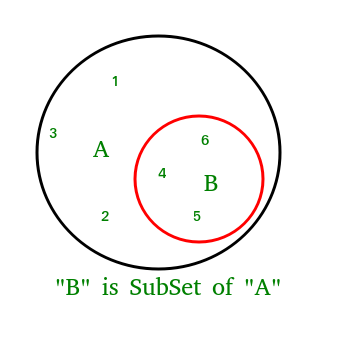Related Articles
issubset() in python
• Last Updated : 06 Feb, 2018

The issubset() method returns True if all elements of a set A are present in another set B which is passed as an argument and returns false if all elements not present.

Syntax:

```A.issubset(B)
checks whether A is a subset of B or not.
Returns:
returns true if A is a subset of B otherwise false.
````# Python program to demonstrate working of ` `# issubset(). ` ` `  `A ``=` `{``4``, ``1``, ``3``, ``5``} ` `B ``=` `{``6``, ``0``, ``4``, ``1``, ``5``, ``0``, ``3``, ``5``} ` ` `  `# Returns True ` `print``(A.issubset(B)) ` ` `  `# Returns False ` `# B is not subset of A ` `print``(B.issubset(A)) `

Output:

```True
False
```

 `# Another Python program to demonstrate working  ` `# of issubset(). ` `A ``=` `{``1``, ``2``, ``3``} ` `B ``=` `{``1``, ``2``, ``3``, ``4``, ``5``} ` `C ``=` `{``1``, ``2``, ``4``, ``5``} ` ` `  `# Returns True ` `print``(A.issubset(B)) ` ` `  `# Returns False ` `# B is not subset of A ` `print``(B.issubset(A)) ` ` `  `# Returns False ` `print``(A.issubset(C)) ` ` `  `# Returns True ` `print``(C.issubset(B)) `

Output:

```True
False
False
True
```

Attention geek! Strengthen your foundations with the Python Programming Foundation Course and learn the basics.

To begin with, your interview preparations Enhance your Data Structures concepts with the Python DS Course.

My Personal Notes arrow_drop_up
Recommended Articles
Page :